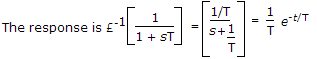# Electronics and Communication Engineering - Automatic Control Systems

### Exercise :: Automatic Control Systems - Section 1

6.

In the given figure shows pole-zero plot. If steady state gain is 2 the transfer function G(s) is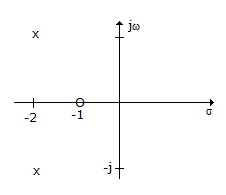A.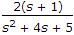B.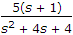C.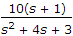D.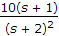Explanation:

The zero at - 1 gives the term (s + 1) in the numerator.

The poles at (- 2 + j1) and (- 2 - j1) give (s2 + 4s + 5) term is the denominator. Gain is 2.

7.

If a system is to follow arbitrary inputs accurately the bandwidth should be

 A. large B. small C. very small D. neither small nor large

Explanation:

Arbitrary inputs require large bandwidth.

8.

For the system of the given figure, the damping ratio of closed loop poles is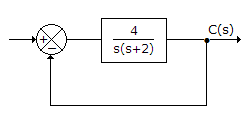A. 1.5 B. 1 C. 0.5 D. 0.25

Explanation:

The standard form of characteristic equation is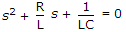.

Therefore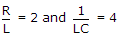.

9.

Assertion (A): Potentiometers can not be used as error detectors in position control systems.

Reason (R): The resolution of a potentiometer places an upper limit on its accuracy

 A. Both A and R are correct and R is correct explanation of A B. Both A and R are correct but R is not correct explanation of A C. A is correct but R is wrong D. R is correct but A is wrong

Explanation:

Potentiometers are used as error detectors.

10.

For a first order system having transfer function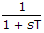, the unit impulse response is

 A. e-t/T B. T e-t/T C.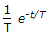D. T2 e-t/T